# SM2国密算法之数学原理

## ECC背后的数学

Posted by 泽群 on November 25, 2020

# 1. SM2算法简介

SM2算法全称是SM2椭圆曲线公钥密码算法（SM是商用密码的拼音缩写），是一种基于“椭圆曲线”的密码。2016年，SM2成为中国国家密码标准。 在商用密码体系中，SM2主要用于替换RSA加密算法。

RSA key size (bits) ECC key size (bits)
1024 160
2048 224
3072 256
7680 384
15360 521

RSA名气很大，并且整数因数分解难题大家感觉自己也能“理解”，但是个人感觉RSA的数学原理比ECC要更加困难，因为RSA的数学原理涉及到许多“数论”的概念，数论不仅抽象，而且不容易理解；而ECC的数学原理刚好是另外一个取向，ECC的数学原理涉及到的数学概念不仅是9年义务教育+高中不会涉及到的，甚至绝大部分专业的大学阶段都也不会接触到，这就使得对于ECC的理解被赋予了很高的门槛，但实际上这些数学概念并没有那么难，并且ECC也只使用了这些数学概念中比较基础的部分，并没有涉及非常深的领域，个人感觉这些概念比数论要容易理解。再加上椭圆曲线是有图像的，图像可以大大帮助我们的理解，所以理解ECC真的没有那么难，你要对自己有信心。

# 2. ElGamal公钥密码体系

SM2作为一种公钥密码算法，自然也需要构造出这么一种单向陷门函数，好消息是，我们并不需要从头开始去“摸索”出这么一种函数，有一种现成且成熟的公钥密码体系——ElGamal公钥密码体系，这种体系基于某些数学概念构造出了一种通用的单向陷门函数，而SM2所基于的椭圆曲线密码正是ElGamal公钥密码体系在椭圆曲线上的应用。

ElGamal体系是构建在有限域的循环子群之上的，SM2的数学基础也是这些，以下内容将介绍这些数学概念。这些数学概念有些抽象，并且比较难于理解，但是这是理解SM2及ECC的必由之路。当你感到困惑时，你要记住，并不是我们要生搬硬套一些抽象的概念，而是有现成的公钥体系建立在这些抽象概念之上的，我们只是想把椭圆曲线应用到这些概念上。

# 3. 什么是椭圆曲线

$\frac{x^2}{a^2}+\frac{y^2}{b^2}=1$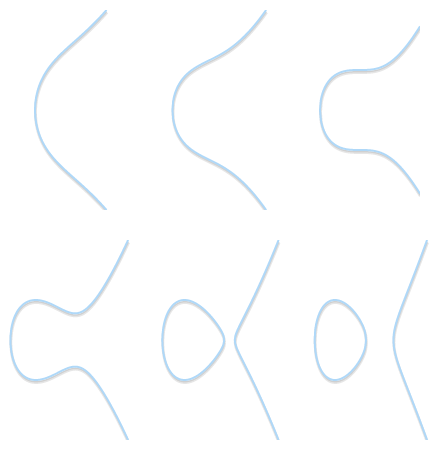# 4. 群论的一点点知识

1. 封闭性：若$a$和$b$是集合$\mathbb{G}$的成员，则$a\cdot b$属于$\mathbb{G}$
2. 结合律：$(a\cdot b)\cdot c=a\cdot (b\cdot c)$
3. 单位元：存在单位元$e$，使得$a\cdot e=a\cdot e=a$
4. 逆元：集合$\mathbb{G}$中的任意成员a，存在b，使得$a\cdot b=e$，a,b互为逆元，记$a=b^{-1}$，同样$b=a^{-1}$。

# 5. 椭圆曲线群

$\begin{array}{rcl} \left\{(x, y) \in \mathbb{R}^2 \right. & \left. | \right. & \left. y^2 = x^3 + ax + b, \right. \\ & & \left. 4a^3 + 27b^2 \ne 0\right\}\ \cup\ \left\{0\right\} \end{array}$

1. 单位元：无穷远点0
2. 三点P,Q,R共线则它们的和为单位元：P+Q+R=0
3. 关于x轴对称的两个点互为逆元。1. 封闭性，显然满足，点加来加去肯定还在椭圆曲线上，要么就是无穷远点。
2. 结合律，满足，三点共线并没有规定顺序，所以(P+Q)+R=0=P+(Q+R)
3. 单位元，就是无穷远点0，每个点加无穷远点还等于这个点
4. 逆元，每个点的逆元肯定存在，因为椭圆曲线关于x轴对称，但是为啥逆元相加等于无穷远点0呢？这个不是很显然，我们可以换个角度看。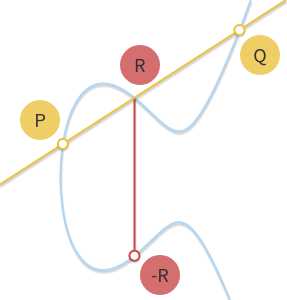1. 两个对称点相加，也就是竖直线与椭圆曲线的只有两个或者一个交点（切线），那么这两个点相加就等于无穷远点0，正如之前所说，没有第三个交点，其实也可以说交点在无穷远处。所以说，逆元相加等于无穷远点0，颇有道理。
2. 相同的点相加，也就是P+P，其实就是取极限，过P点椭圆曲线的切点，见图。
3. P为切点，P+Q=-P，一样取极限，见图。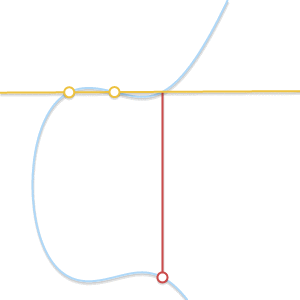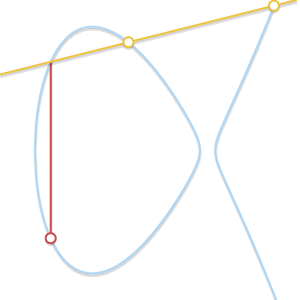## 5.1 标量乘法

$nP = \underbrace{P + P + \cdots + P}_{n\ \text{times}}$

$151 = 2^7 + 2^4 + 2^2 + 2^1 + 2^0$

$151P = 2^7P + 2^4P + 2^2P + 2^1P + 2^0P=128P+16P+4P+2P+P$

# 6. 有限域

$(18+9) \pmod{23} = 4 \\ (7-14) \pmod{23} = 16 \\ 4\times 7 \pmod{23} = 5 \\$

$\frac{1}{9}\pmod{23} = 9^{-1} \pmod{23}$

$9\times ? \pmod{23}=1(1是乘法的单位元)$

$\frac{1}{9}\pmod{23} = 9^{-1} \pmod{23}=18$

# 7. 有限域上的椭圆曲线

$\begin{array}{rcl} \left\{(x, y) \in (\mathbb{F}_p)^2 \right. & \left. | \right. & \left. y^2 \equiv x^3 + ax + b \pmod{p}, \right. \\ & & \left. 4a^3 + 27b^2 \not\equiv 0 \pmod{p}\right\}\ \cup\ \left\{0\right\} \end{array}$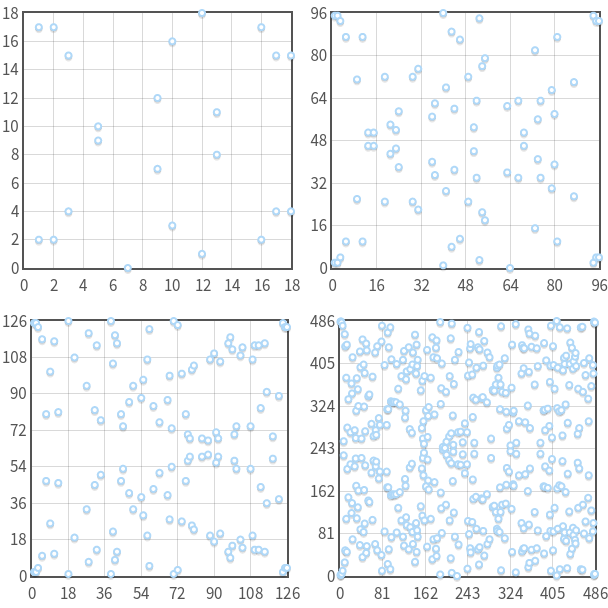## 7.2 循环子群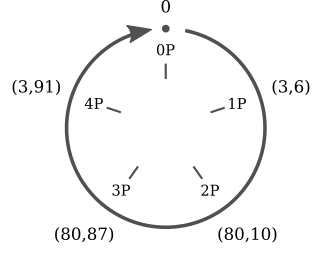$5P=0$，并且你可以继续验证$6P=P,7P=2P \dots kP = (k \bmod{5})P$。这五个点组成了一个封闭集合，你在这个集合中做点加运算，结果仍在在这个集合中，我们称这个集合是群的一个循环子群。对于上例而言，该循环子群的阶就是5，这个循环子群中的其它点都是通过点P得到的，我们称P是这个循环子群的基点或者生成元

# 8. 椭圆曲线离散对数难题

ECC的安全性建立在椭圆曲线离散对数难题（elliptic curve discrete logarithm problem，ECDLP）之上的，这个问题之所以困难，并不是说我在这嘚啵了半天，引入了这么多数学概念它才困难，而是它真的困难（拜托，相信我）——这个理由还真的是一点都不牵强啊！关键还在于，我们找不出有效的算法来求解这个问题，总之，你知道这是个难题就可以了。

# 9. 椭圆曲线密码学ECC

1. ECDH（Elliptic Curve Diffie-Hellman）：密钥交换
2. ECDSA（Elliptic Curve Digital Signature Algorithm）：数字签名
3. 非对称加密

## 9.1 密钥交换

• 有限域上的椭圆曲线基点为$G$
• Alice的私钥是$d_A$,公钥是$H_A=d_AG$
• Bob的私钥是$d_B$,公钥是$H_B=d_BG$
• Alice和Bob相互交换公钥，他们可以求出相同的密钥S

$S = d_A H_B = d_A (d_B G) = d_B (d_A G) = d_B H_A$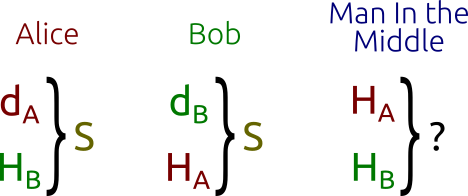## 9.2 数字签名

• 用密码级安全的哈希函数把待签名的信息m转换为哈希值z
• 在${1, \dots, n - 1}$范围内生成随机数k，n为循环子群的阶
• 计算$P = kG$
• $r = x_P \bmod{n}\ (x_P是P的横坐标)$
• $s = k^{-1} (z + rd_A) \bmod{n}$
• (r,s)就是签名信息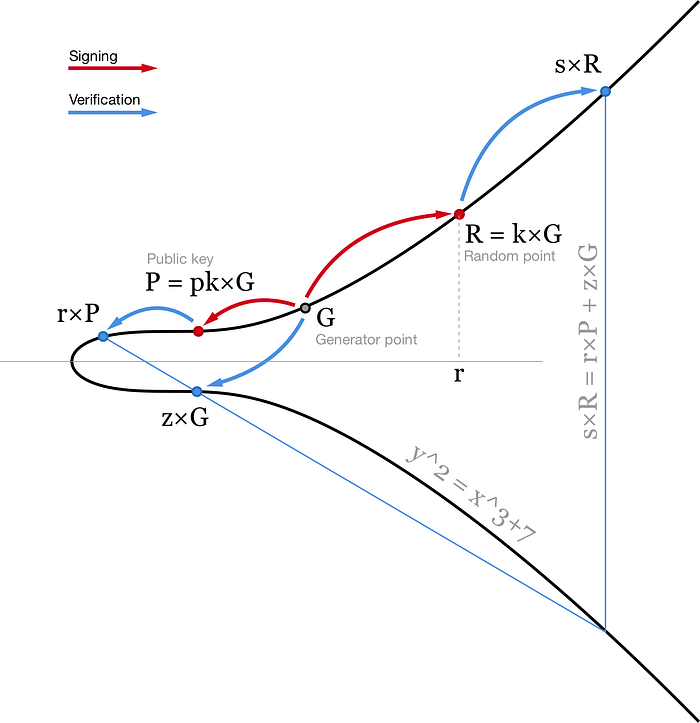s之所以设计成那个样子就是为了使得，rP+zG=sR。

## 9.3 非对称加密

• Alice的私钥是$d_A$，公钥是$H_A=d_AG$
• Bob将要发送的消息m编码为椭圆曲线上的点M，并生成一个随机数r
• Bob把$C_1=M+rH_A \ \ C_2=rG$ 发给Alice
• Alice解密$C_1-d_AC_2=M+rH_A-d_ArG=M+rd_AG-d_ArG=M$

# 10. 标准曲线

secp256k1标准曲线的领域参数如下：

1
2
3
4
5
6
7
p = 0xFFFFFFFFFFFFFFFFFFFFFFFFFFFFFFFFFFFFFFFFFFFFFFFFFFFFFFFEFFFFFC2F
a = 0
b = 7
xG = 0x79BE667EF9DCBBAC55A06295CE870B07029BFCDB2DCE28D959F2815B16F81798
n = 0xFFFFFFFFFFFFFFFFFFFFFFFFFFFFFFFEBAAEDCE6AF48A03BBFD25E8CD0364141
h = 1


SM2标准椭圆曲线叫做sm2p256v1，具体领域参数如下：

1
2
3
4
5
6
7
p = 0xFFFFFFFEFFFFFFFFFFFFFFFFFFFFFFFFFFFFFFFF00000000FFFFFFFFFFFFFFFF
a = 0xFFFFFFFEFFFFFFFFFFFFFFFFFFFFFFFFFFFFFFFF00000000FFFFFFFFFFFFFFFC
b = 0x28E9FA9E9D9F5E344D5A9E4BCF6509A7F39789F515AB8F92DDBCBD414D940E93
xG = 0x32C4AE2C1F1981195F9904466A39C9948FE30BBFF2660BE1715A4589334C74C7
yG = 0xBC3736A2F4F6779C59BDCEE36B692153D0A9877CC62A474002DF32E52139F0A0
n = 0xFFFFFFFEFFFFFFFFFFFFFFFFFFFFFFFF7203DF6B21C6052B53BBF40939D54123
h = 1


# 11. SM2在设计什么

1. 选择合适的领域参数，避免“弱曲线”，对抗已知的攻击手段。
2. 设计出具体的密钥交换、数字签名、非对称加密的标准算法，即要保证安全，又要保证计算效率。

# 12. 问题

1. 悖论：标准椭圆曲线是否暗藏后门，椭圆曲线离散对数问题并不是在任何条件下都是困难的，在某些特定的椭圆曲线上就比较简单，那么如果保证标准的椭圆曲线不是经过“精心设计”的，然后设计者知道某些不公开的破解手段呢？这是个悖论，无论标准椭圆曲线的参数取什么值都会有这样的疑虑。相反RSA就不存在这样的问题，因为也不需要什么标准的素数这种东西。但是，我觉得这也不是个问题，想通过“公开”的方式去“隐藏”这本身就不太可行。
2. 信息如何编码到点，如何从点反推出信息，这部分内容没有涉及，因为我也不知道。
3. 椭圆曲线有很多种，文中所说的都是一种叫Weierstrass的椭圆曲线，这种椭圆曲线最常用，当然还有别的形式的椭圆曲线。
4. 常见的有限域除了$F_p$还有$F_2^m$，$F_p$上的运算比较好理解，$F_2^m$上的运算就不是那么容易理解了，当然，也有定义在$F_2^m$有限域上的标准曲线。

# 14. 参考

How Schnorr signatures may improve Bitcoin，这篇文章介绍了比特币签名的细节，本文ECDSA的图片即来自这篇文章。